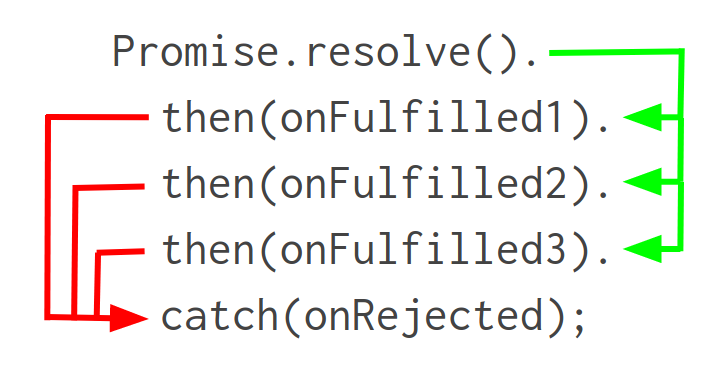# JavaScript Promise Chaining

Apr 15, 2020

Promise chaining is what makes promises meaningfully better than callbacks. The key idea is that a Promise's `then()` function returns another promise, so you can chain `.then()` calls together to tell JavaScript to execute async calls in order.

``````const start = Date.now();
return Promise.resolve().
then(() => new Promise(resolve => setTimeout(resolve, 50))).
then(() => new Promise(resolve => setTimeout(resolve, 50))).
then(v => {
console.log(Date.now() - start); // About 100ms passed
});``````

## Return Values

The first parameter to the `then()` function is called `onFulfilled()`. That's because JavaScript calls that function when the promise is fulfilled. JavaScript calls the `onFulfilled()` function with the value the promise was fulfilled with as the first parameter.

Promise chaining works because, if your `onFulfilled()` function returns a promise `q`, the promise `then()` returns will adopt the state of `q`. So the promise `then()` returns will have the same fulfilled value as `q`.

``````return Promise.resolve(1).
// If `onFulfilled()` returns a promise, JavaScript calls the
// next `onFulfilled()` with the fulfilled value of the promise
then(v => new Promise(resolve => setTimeout(() => resolve(v + 1), 10))).
then(v => new Promise(resolve => setTimeout(() => resolve(v + 1), 10))).
then(v => new Promise(resolve => setTimeout(() => resolve(v + 1), 10))).
// If `onFulfilled()` returns a value that isn't a promise,
// JavaScript calls the next `onFulfilled()` with that value.
then(v => v + 1).
then(v => {
console.log(v); // 5
});``````

## Error Handling

Promise chaining also consolidates error handling. All you need is one `.catch()` function call at the end of your promise chain to handle any errors that occur in your promise chain.

``````Promise.resolve(1).
then(v => v + 1).
// Async error in the middle of the chain goes straight
// to `catch()`.
then(() => Promise.reject(new Error('Oops'))).
then(v => v + 1).
catch(err => {
err.message; // 'Oops'
});

Promise.resolve(1).
then(v => v + 1).
// Sync error in the middle of the chain goes straight
// to `catch()` too.
then(() => { throw new Error('Oops'); }).
then(v => v + 1).
catch(err => {
err.message; // 'Oops'
});``````

## Summary

The high level structure of a promise chain is a series of `.then()` calls, each with an `onFulfilled()` parameter, and a single `.catch()` at the end. JavaScript executes the `.then()` callbacks in order, or goes straight to `.catch()` if one of the `onFulfilled()` functions errors out.Did you find this tutorial useful? Say thanks by starring our repo on GitHub!